# 【线性回归类算法的建模与评估】1.多元线性回归

2.Lasson回归

3.岭回归

1.预测函数

2.预测值向量

4.损失函数

5.最小二乘解

6.数据多线性

7.多元线性回归正则化

1.均方误差

2.决定系数

1.模型选择

2.调参

3.训练集和测试集

4.提交用户模型

1.准备数据

2.测试代码

3.测试结果

## 2.Lasson回归

lasso回归只起到一个筛选变量得作用，用完之后还得使用原来的线性回归。

## 1.预测函数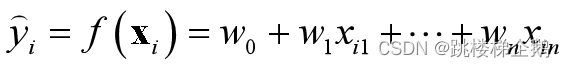## 2.预测值向量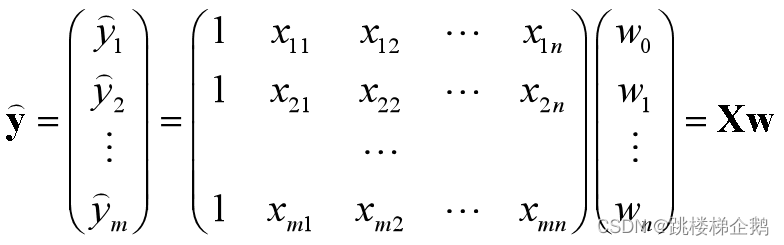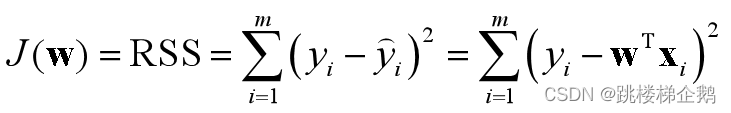## 4.损失函数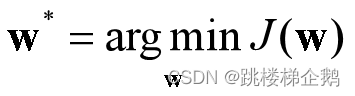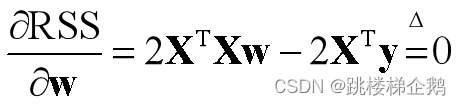## 5.最小二乘解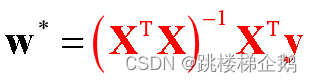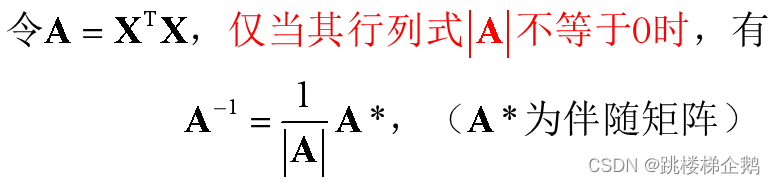行列式在经过初等行/列变换后，其值保持不变。可以先变换成上或下三角形状，再求对角线元素之积。若一个矩阵变换为上(下)三角矩阵后，对角线元素全都不为零，则称为满秩矩阵，此时对应行列式值不为零，故可逆。

## 6.数据多线性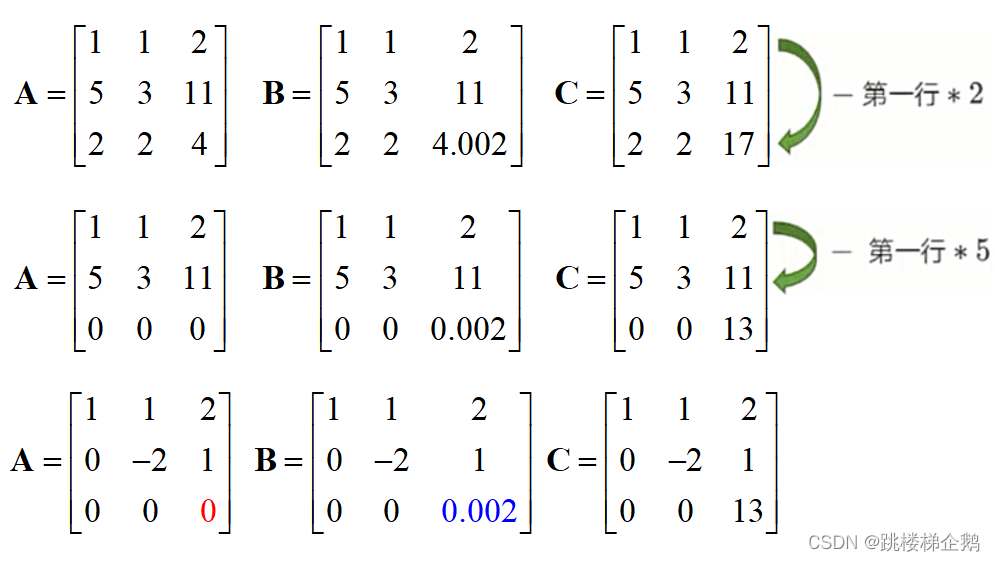（1）矩阵中某些行出现精确相关时，行列式值等于0，最小二乘法无法使用，无法求出线性回归结果。 （2）矩阵中某些行出现高度相关时，上三角变换后出现值非常接近0的对角线元素，使得行列式值接近0，最小二乘法求得的参数将会很大，影响建模结果，导致模型偏差过大，或不可用。 （3）上述精确相关和高度相关并称为多重共线性(multicolinearity)

## 7.多元线性回归正则化

（1）在线性回归算法基础上进行修改，使其能容忍特征列存在多重共线性的情况，并且能顺利建模，且尽可能保证RSS最小。 （2）岭(ridge)回归、Lasso(最小绝对收缩和选择算子：least absolute shrinkage and selection operator)回归、弹性网(elastic net)回归在线性回归损失函数的基础上分别添加一个L2范数正则项、 L1范数正则项、 混合正则项。 （3）这些算法实际使用时，模型效果往往反倒会下降一些，可能是因为这些算法不是为了提升模型表现，而是为了修复线性回归不能处理多种共线性这一漏洞而设计的，而且它们还挤占了原本参数估计的信息空间。由于线性回归模型偏向于欠拟合，因而很少需要考虑过拟合问题，反倒L1正则化更多地用于进行特征选择。

（4）正则化(Regularization)：带正则项和带约束条件是等价的。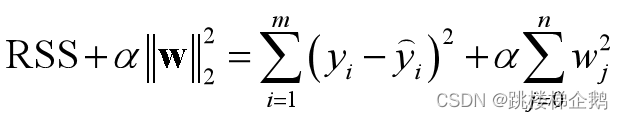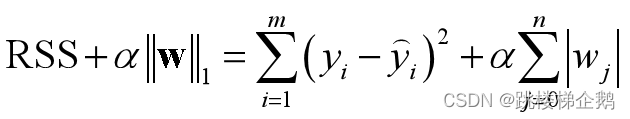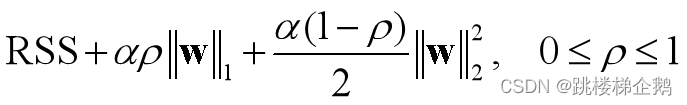（5）正则化不仅仅适用于线性回归算法。原理上说，L1范数代表了向量中各元素绝对值之和，可以实现稀疏和特征选择；L2范数可看成是向量的模，能避免过拟合。

线性回归模型的特点是自变量(特征)都是一次项，适合用来拟合线性数据(即标签与特征之间呈近似线性关系)，而且具有计算速度快的明显优势。     使线性回归在非线性数据上表现提升的一个重要方法就是使用多项式转换升维(增加特征数)来改进线性回归：多项式转换通过对自变量(特征)进行幂次运算并进行组合，产生大量组合特征，从而将数据映射到高维空间。

从两个角度评价回归效果：预测数值的准确度（MSE和MAE）；对数据提供的信息和内在规律的拟合程度（R2）。

## 1.均方误差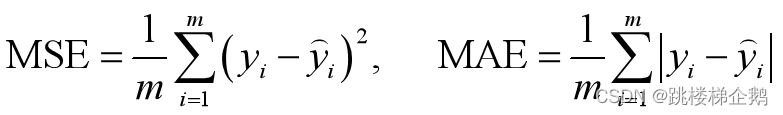## 2.决定系数

决定系数(coefficient of determination)R2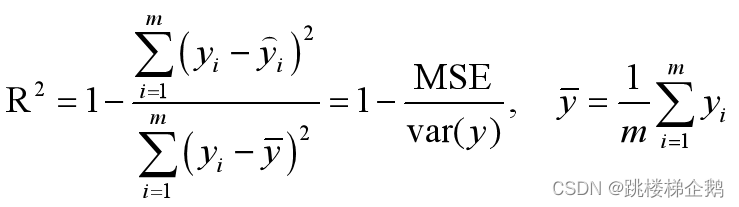R2表示模型捕获到的信息量占真实标签中所带信息量的比例，因此越接近1越好。

## 1.准备数据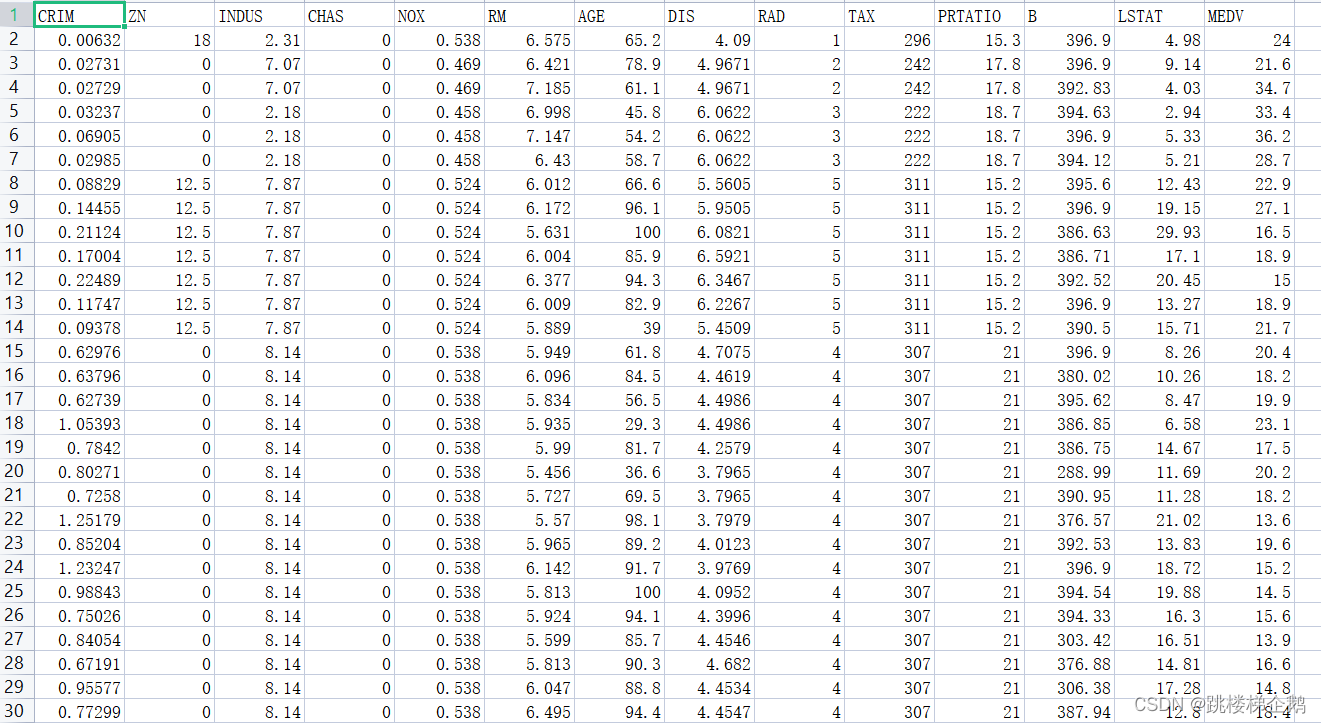## 2.测试代码

``````#%%1.读入数据并划分训练集和测试集

import pandas as pd

from sklearn.model_selection import train_test_split

X = data.iloc[:,0:13]

y = data.iloc[:,13]

Xtrain,Xtest,ytrain,ytest = train_test_split(

X,y,test_size=0.2,random_state=520)

#%%2.在训练集上，对线性回归、岭回归、Lasso回归、弹性网回归四种算法进行交叉验证评估，指标是MSE和R2。

from sklearn.linear_model import LinearRegression as LR

from sklearn.linear_model import Ridge,Lasso,ElasticNet

models={}

models['LR'] = LR()

models['Ridge'] = Ridge()

models['Lasso'] = Lasso()

models['EN'] = ElasticNet()

results = {}

from sklearn.model_selection import cross_val_score

for key in models:

mse_cv = cross_val_score(models[key],

Xtrain,ytrain,cv=5,scoring="neg_mean_squared_error").mean()

r2_cv = cross_val_score(models[key],

Xtrain,ytrain,cv=5,scoring="r2").mean()

results[key] = [-1*mse_cv,r2_cv]

print(pd.DataFrame(results,index=["mse_cv","r2_cv"]))

#%%在训练集上建模并在测试集上比较多元线性回归和多项式回归的R2评分，然后进行预测结果可视化。

from sklearn.preprocessing import PolynomialFeatures as PF

from sklearn.metrics import r2_score

reg = LR()

reg.fit(Xtrain,ytrain)

yhat = reg.predict(Xtest)

r2 = r2_score(ytest,yhat)

##多项式回归建模

poly = PF(degree = 2,include_bias=False)

Xtrain_ = poly.fit_transform(Xtrain)

Xtest_ = poly.fit_transform(Xtest)

reg_ = LR().fit(Xtrain_,ytrain)

yhat_ = reg_.predict(Xtest_)

r2_ = r2_score(ytest,yhat_)

print("二次多项式回归模型在测试集上的R2的评分",r2)

#%%4.进行预测可视化

import matplotlib.pyplot as plt

plt.plot(range(len(ytest)),sorted(yhat),linewidth=2,color='green'

,label = "ploy regression")

plt.plot(range(len(ytest)),sorted(yhat_),linewidth=2,color='red'

,label = "ploy regression")

plt.plot(range(len(ytest)),sorted(ytest),linewidth=2,color='blue'

,label = "ploy regression")

plt.legend()

plt.show()``````

## 3.测试结果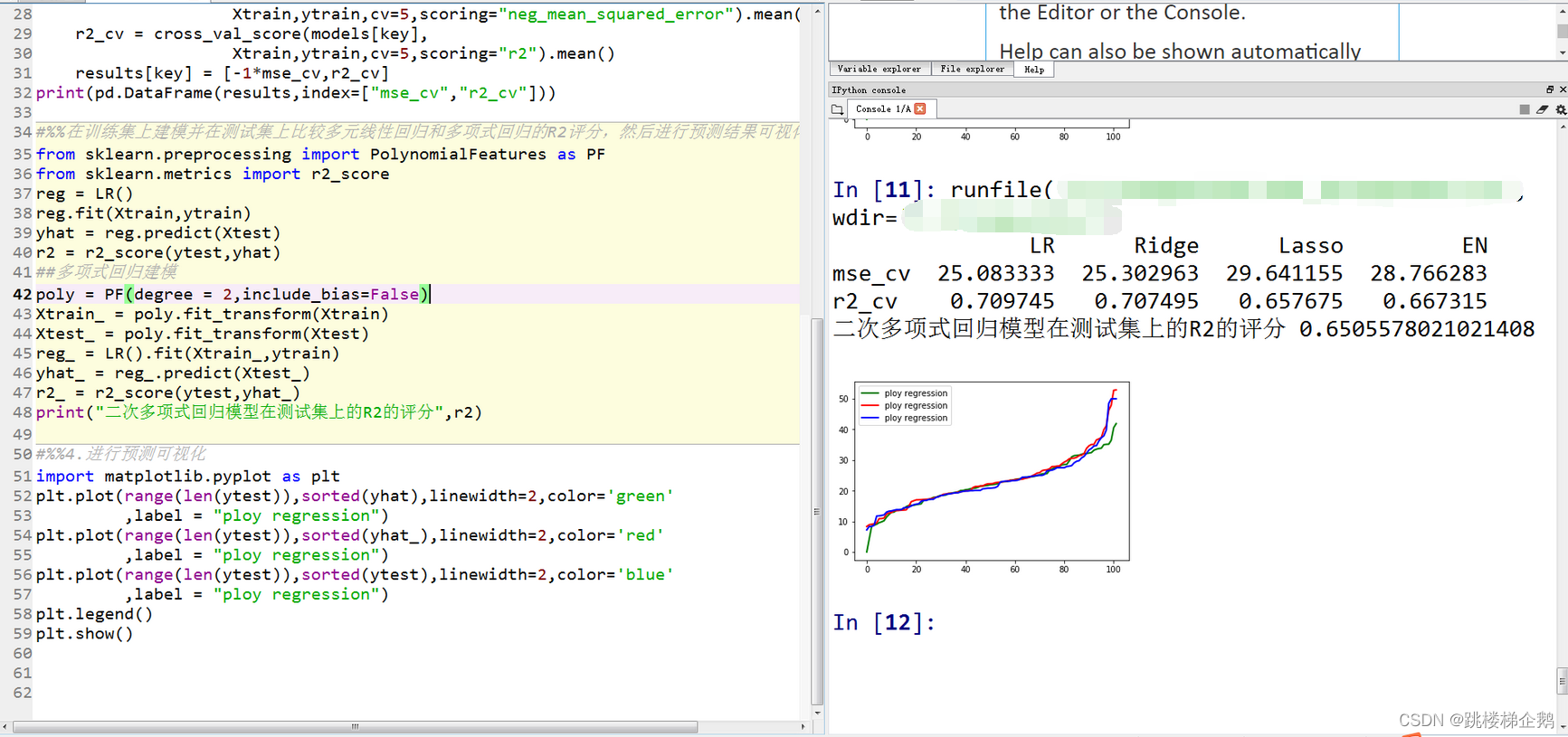-- 展开阅读全文 --

BUUCTF Web [极客大挑战 2019]Knife
« 上一篇 06-24